• Submissions# Radiative Heat Transfer in the Chemically Reactive Unsteady MHD Squeezed Flow of Second Order Fluid with Heat Source

Siddiqui AM1, Maqbool K2, Ayesha Sohail3 and Mann AB4*

1Department of Mathematics, Pennsylvania State University, USA

2Department of Mathematics & Statistics, International Islamic University, Pakistan

3Department of Mathematics, COMSATS Institute of Information Technology, Pakistan

4Department of Mathematical Sciences, Federal Urdu University of Arts, Science & Technology, Pakistan

*Corresponding author: Mann AB, Department of Mathematical Sciences, Federal Urdu University of Arts, Science & Technology, Near Zero Point, G-7/1, Islamabad 44000, Pakistan

Submission: December 10, 2017; Published: March 27, 2018

DOI: 10.31031/RDMS.2018.04.000598ISSN: 2576-8840
Volume4 Issue5

#### Abstract

This study investigates the radiative heat and mass transfer effects on squeezed flow of unsteady MHD second order fluid with heat source and chemical reaction. This study is carried out between two parallel circular plates under the assumption of axisymmetric squeezed flow. Similarity transform method is used to reduce the system of partial differential equation (PDE’s) into the system of ordinary differential equations (ODE’s). Advanced Collocation Method is used to find the solutions of nonlinear ordinary differential equations for velocity profile, pressure distribution, and temperature and concentration field. Effects of pertinent physical parameters on the computational results for the radial and axial velocity, pressure distrinution, and temperature and concentration profile are presented via graphs. The shear stress, heat transfer rate and mass transfer rate on the upper wall are also shown by the help of graphs.

Keywords: Heat source; Chemical reaction; Thermal radiation; Advanced collocation method, Second order fluid; MHD squeezing flow

Nomenclature: B: Total Magnetic Field; J: Current Density; M: Hartmann Number; P: Pressure; Q: Heat Source Parameter; R: Chemical Reaction Parameter; R: Chemical Reaction Parameter; S: Squeezed Number; Pr: Prandtl Number; Rd: Radiation Parameter; Sh: Local Sherwood Number; Sc: Schmidt Number; h(t): Speed of the Upper Plate; u, w: Velocity Components; r, z: Cylindrical polar coordinates; t: Time; ϕ: Dimensionless Concentration; Ƞ: Similarity Variable; ɵ: Dimensionless Temperature; α1¬, α2: Second Grade Fluid Parameters; ϒ: Thermal Slip Parameter

#### Introduction

It is a critical issue of modern world to develop the new techniques for renewable energy. The main sources of renewable energy are solar energy, hydropower, fossil fuel and wind. The heat and electric energies can be produced by the natural source in the form of solar radiation and solar powers. Moreover, radioactive heat transfer play a vital role in science and technology such as heating and cooling chamber, rivers, canals, dams, food processing, textile engineering and many other industrial processes. Many researchers [1- 5] are developing new energy sources and technologies of renewable energy. In addition, most of the computer system and electrical powers dissipate the heat energy therefore heat source and sink are important for the reliable performance of electronic operators. For a number of heat source/sink problems studies are investigated by a number of researchers [6,7]. Chemical engineering requires new techniques consisting of material manufacture and polymers in bioreactors in medical biotechnology.

The dynamics of biofluids exhibit the characteristics of thermofluid for which the mass transfer is the vital agent of bioreactor design. The fluid dynamics of such system shows the heat and mass transfer effects in confined boundaries such as fire dynamics, fuel cells and solar cell technologies. Effect of chemical reaction and heat source on heat and mass transfer have been discussed by various investigators, e.g. Muthucumaraswamy et al. [8,9] studied the unsteady upward flow of viscous fluid with chemical reaction and heat source on an isothermal and oscillating plate. Saxena SS & Dubey GK  discussed the effect of heat and mass transfer for the flow of viscoelastic fluid with heat source, thermal radiation and slip boundary conditions. Ibrahim et al. [11,12] discussed the impact of heat source and chemical reaction on viscous fluid passed through a porous regime with Joule heating and viscous dissipation later he has extended these effects on unsteady MHD flow of viscoelastic fluid. Noreen SA et al.  discussed the radiative heat and chemically reactive mass transfer of MHD peristaltic flow of Maxwell fluid in a symmetric channel through a porous medium. The work of Hayat et al.  analyzed the unsteady axisymmetric squeezed flow of Jeffrey fluid between parallel plates under the radiation effect. The heat and mass transfer analysis of MHD second grade fluid in the presence of thermal radiation and diffusion is carried out by Olajuwon et al. . Recently, group theoretical method to study the similarity solution of convective heat and mass transfer flow for MHD Newtonian fluid with convective boundary conditions is studied by Rashidi et al. [16,17] also studied thermal convective boundary conditions and hydrodynamic slip conditions of convective heat and mass transfer flow along a plate kept in vertical direction.

Squeeze flows may observe between two parallel rectangular or circular plates; however the squeeze motion depends on the geometry of the surface. In squeeze flows solid or liquid is compressed between two disks and is thus squeezed out. Squeeze flows are investigated in different field of research e.g. geophysics, material science, biotechnology, engineering science and fluid mechanics.

In 1874 Stefan et al.  first considered reverse squeeze flows for the Newtonian fluid, later in 1886, Reynolds  obtained the results for the predictable squeezed flow for the Navier Stokes equation under the lubrication approximation. Scott  extended the Reynolds’ idea for the non-Newtonian fluid showing the shear thinning and thickening effects for the creeping flow. It is analyzed from the literature review that analytic solutions for axisymmetric and plane squeezed flow for viscous and viscoelastic fluids are discussed by various researchers [21-29]. In addition some other researchers studied the thermo dynamic effects of squeezed flow like Khaled & Vafai  discussed heat transfer effect for the squeezed flow of viscous fluid on a sensor surface. Mahmood et al.  presented heat transfer analysis of squeezed flow on a porous surface. Squeezed flow and heat transfer analysis between two plates was considered by Duwairi et al. . Recently, Mustafa et al.  addressed the heat and mass transfer of squeezing flow of Newtonian fluid through a rectangular channel. Khan et al.  has compared the analytic solution of viscous fluid for the axisymmetric flow in a slip regime. Ganji et al.  discussed the analytic solution of squeezed flow with magnetic effects between two porous plates by Homotopy analysis method, he has also compared the results for suction and blowing cases by Homotopy perturbation method.

The broad aim of present work is to analyze the radiative heat and mass transfer flow of MHD second order fluid between two parallel discs with chemical reaction and heat source subjected to squeezing system. The similarity transform method is used to transform the system of partial differential equations into ordinary differential equations. Numerical technique (Advanced collocation Method) is used to solve the ordinary differential equations governing the fluid flow, pressure distribution, concentration and temperature profile.

#### Problem Formulation

Let us consider an unsteady axis symmetric squeezing flow of second order fluid between two parallel circular plates moving with speed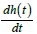and distance between both plate iswhere is the characteristic time parameter. A constant magnetic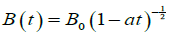is applied transverse to the plates. The induced magnetic field can be neglected due to the small magnetic Reynold’s number. The plates move symmetrically about radial direction while flow is axisymmetric about the axial direction. The temperature and mass concentration on the lower plate are T0 and C0 while Th and Ch are temperature and concentration on the upper plate as shown in Figure 1.

Figure 1:Schematics diagram of the problem.The governing mass, momentum, energy and diffusion laws for second order/grade fluid can be stated as

divV = 0, (1)where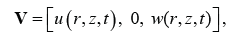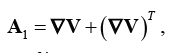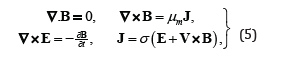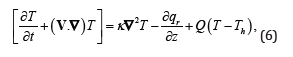whereandThe B.C’s on velocity, temperature and concentration profile arewhere is the radial velocity, w is the axial velocity, C and are the concentration and temperature profile respectively.ρ , μ, σ , κ , Dm Qand R are the density of the fluid, viscosity of the fluid, electrical conductivity, thermal diffusivity, diffusivity constant, heat source parameter and chemical reaction parameter respectively.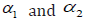are second order/grade fluid parameters, 1 A is the first RivlinEricksen tensor J is the current density ε is the electric field and due to no polarization effect E = 0, σ is the Stefan constant and km mean absorption coefficient and γ is thermal slip parameter.

Let us define following parameters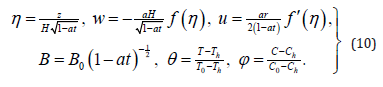In view of equation (10) the governing laws and boundary conditions in Eqs.(1)-(9) reduce tosubject to the B.C’swhere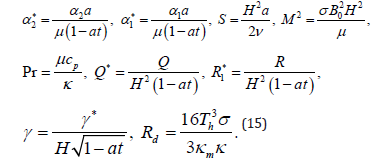In order to find the pressure distribution using Eq. (10) in Eqs. (2)-(5), we get the following expressionwhere P is the modified pressure and P0 is the reference pressure.

The pressure on the upper plate is given in the following formThe important characteristics of velocity, heat and mass transfer are the skin friction coefficients , cfrh Nusselt number Nu and Sherwood number Sh and are defined as followsThe wall shear stress, skin friction coefficient, Nusselt number and Sherwood number in non-dimensional form are given by the following expressionswherein whichdenotes the local Reynolds number, Cfr is the skin friction coefficient at the upper plate, Nur is the heat transfer rate on the upper plate and Shr is the mass concentration rate on the upper plate.

#### Numerical Solution

We have successfully transformed the governing partial differential equations (PDE’s) to ordinary differential equations (ODE’s) with the use of similarity transform method. We have solved the transformed equations (11-14) subject to boundary conditions in Eq. (14) numerically using an advanced collocation method. This mathod is useful because, it reduces the nth order differential equation(s) into first order differential equations, thus reducing the computational cost on a large domain with small step size and a range of parameters. We have simplified the system of equations (11-14) using the Modified Collocation Method (CM). The use of polynomial or piecewise polynomial collocation spaces for the approximate solution of BVP has its origin in the 1930s. Khater et al.  much later elaborated a spectral collocation method (SCM) based on differentiated Chebyshev polynomials to find numerical solutions for nonlinear partial differential equations (PDE’s). They reduced the nonlinear equations to the system of ordinary differential equations and solved by Runge--Kutta Method of order four (RK4).

Therefore the key role of this method is that, it reduces the order differential equation(s) into first order differential equations, thus reducing the computational cost on a large domain with small step size and a range of parameters. We have simplified the system of equations (11)-(14) using the Modified Collocation Method (CM). The collocation solver  when applied to the BVP produces continuous solution on an appropriate mesh.

For a general BVP defined on the interval [x0,xm] subject to two-point boundary conditions, bvp4c implements a Collocation Method (CM) for the solution of Boundary Value Problems of the form:subject to nonlinear B.C’swhere p is a vector of unknown parameters. The numerical solution W(x) encompasses a continuous functions which is a polynomial of order three on each subinterval [xi,xi+1] of the finite difference grid (mesh) defined by:satisfying the associated boundary conditions.

Furthermore it satisfies the nodes at both (left and right) ends and the middle of each sub-interval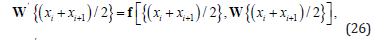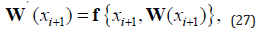The above conditions generate a robust family of non-linear algebraic equations for the coefficients defining W(x) . Distinct from shooting algorithms, the solution y(x) is approximated on the domain [x0, xm] The boundary conditions are considered at all stages of the computations. The nonlinear algebraic equations are iteratively solved via linearization and this is achieved via the linear equation solvers in MATLAB, and not the IVP solvers. Essentially the bvp4c algorithm is a modified Simpson stepping scheme and W(x) is a fourth order approximation to an isolated solution y(x) , viz: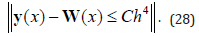Here designates the maximum number of step sizes hi = xi+1-xi , and C is a constant. This bound is enforced for all x in [x0, xm] . Following computation of W(x) on the mesh with bvp4c, it is updated and improved quickly and cost-effectively at any x or set [x0, xm].

To be more precise, the obtained collocation solutions to the system of ordinary differential equations (11-14). Thus we used a finite difference code that construct a collocation formula and the collocation polynomial gives a continuous solution in the domain . The Iterative method is used to solve the linearized nonlinear algebraic equations, so this method relies upon Matlab solver of the linear equation. The choice of max η =1 guaranteed that all numerical solutions approached the asymptotic values correctly, although the finite choice of max η will be not fully responsible for minimized order of accuracy, such as Kuznetsov & Nield  & Sohail et al.  compared their results for the special case of a regular fluid with their numerical results obtained by keeping max η =10 , and ignored the error generated by limiting the domain, in a similar manner, we will ignore that error.

#### Numerical Results and Discussion

Here we discussed the effect of pertinent parameters on the velocity profile, temperature profile, concentration profile skin friction coefficient, local Nusselt number and local Sherwood number.

Figure 2:Influence of squeezing parameter S on f (η ) and f ′(η )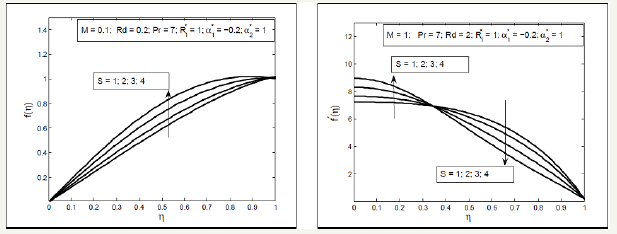Effect of Squeezed number S on the radial and axial velocity f ′(η ) and f (η ) have been analyzed through Figure 2. It is analyzed that the radial velocity f ′(η ) decelerate near the upper plate and enhances near the lower plate and axial velocity f (η ) accelerate between two plates with the increase in Squeezing parameter S . Effects of magnetic parameter can been seen through Figure 3, it is depicted that the axial velocity f (η ) slow down with the increase in Hartmann number M but the radial velocity shows the f ′(η ) dual behavior first decreasing then increasing. In fact the magnetic field acts along the axis that resist the fluid motion consequently the axial velocity decreases also the radial velocity near the lower plate decelerate due to Lorentz force but near the above plate the squeezing effects are dominant therefore the velocity increases. Figure 4 display the effects of fluid parameters It is seen from the figures that increasing values of parameters causes to increase the viscosity of the fluid so the axial velocity decreases but the radial velocity near the upper plate enhances as the upper plate moves towards the lower stationary plate. Variation of thermal slip parameter γ and squeezed number S on the temperature profile is shown in Figure 5. It is observed that temperature θ (η ) profile enhances near the lower plate and decreases near the upper plate for higher thermal slip parameter. It is due to the fact that the lower plate provides thermal conductivity whereas the upper plate provides large amount of thermal resistance which consequently decelerate the temperature distribution. The higher value of squeezing parameter shows the increase in temperature profile away from the lower plate but on the lower plate temperature profile decreases due to thermal slip. Increasing values of squeezing parameter illustrate that viscosity decreases as the distance between two plate’s increases. Figure 6 is plotted for different values of Prandtle number Pr and heat source parameter (Q ) to illustrate the impact of Pr and Q on temperature profile. It is observed that increasing value of Pr causes to reduce the thermal conductivity of the fluid away from the lower plate due to which the temperature profile decreases. The increasing value of heat source parameter Q causes to accelerate the rate of heat transfer thus it enhances the temperature profile. Figure 7 shows the impact of magnetic parameterM and radiation parameter d R on temperature profile, it is observed that temperature profile decrease with the increase in magnetic parameter as it resist the motion of the fluid so the fluid become thick and thermal conductivity decreases so the temperature profile decelerate while the radiation parameter enhances the heat transfer. Figure 8 show that the decrease in fluid parameter thermal conductivity near the lower increases therefore the temperature profile increases but near the upper plate thermal conductivity reduces so the temperature profile decelerate also the concentration profile near the lower plate increases due to increase in Schmidt number and decreases near the upper plate i.e. viscous diffusion rate enhances the mass concentration near the lower plate but near the upper plate the squeezed effect are strong as compared with the viscous diffusion rate therefore mass concentration decelerate Figure 9 shows the influence of chemical reaction parameter ( 1 R ) and squeezing parameter ( S ) on the concentration profile. It is depicted from the plots that the chemical reaction parameter causes to increase the mass concentration of the fluid also squeezing parameter show the increasing behavior of the concentration profile.

Figure 3:Influence of Hartmann number M on f (η ) and f ′(η )Figure 4:Influence of second grade parameter 1 a ∗ on f (η ) and f ′(η )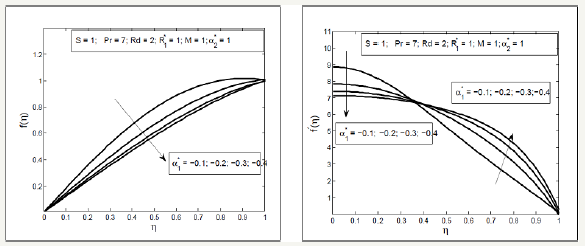Figure 5:Influence of thermal slip parameter γ and squeezing parameter S and θ (η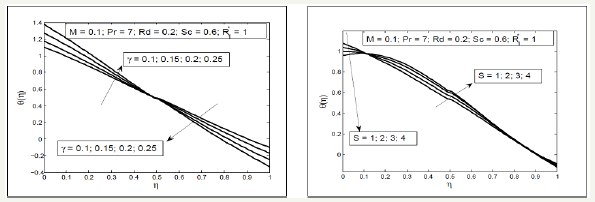Figure 6:Influence of Prandtl number Pr and heat source parameter on θ (η ).Figure 7:Influence of Hartmann number M and radiation parameter Rd on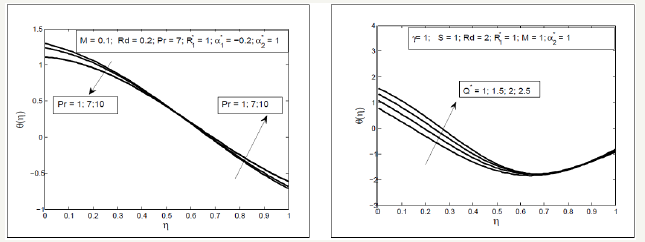Figure 8:Influence of second order parameter on θ (η ). and Sc on ϕ (η ).Figure 9:Influence of chemical reaction parameter R1* and squeezing parameter S on ϕ (η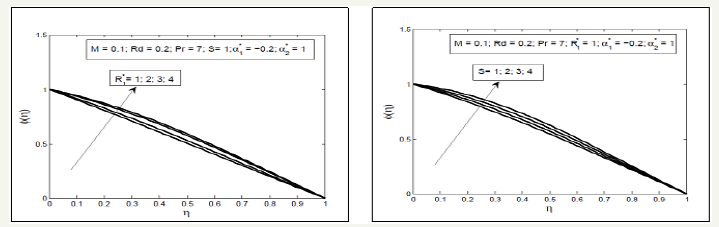Figure 10:Influence of Hartmann number M on skin friction coefficient Cfrand chemical reaction parameter R1*on Schmidt number SC.Figure 11:Influence of heat source Q∗ and thermal slip parameter γ on Nusselt number Nu.Figure 12:Influence of fluid parameter a1* and squeezing parameter S on pressure at the upper plate..The skin friction plays an important role in thermal and engineering processes, since it is the friction between the surface of a moving solid through a fluid and the fluid itself. It is depicted from Figure 10 & 11 that skin friction at the upper plate increases with the increase in magnetic parameter while for large value of squeezing parameter skin friction decreases. Nusselt number ( Nu ) is defined as the rate of heat transfer at the surface when a fluid past through a solid surface. It is observed that the Nusselt number shows the increasing behavior with the increase in heat source parameter Q but it decreases with the increase in thermal slip parameter also Nusselt number increases for the large values of Prandtl number. Further, it is seen that the Sherwood number ( Sh ), is the rate of mass transfer at the surface and it decreases for the large values of chemical reaction and increases for large Schmidt number.

Figure 12 illustrate that the pressure on the upper plate increases in negative direction with the increase in the fluid parameter and squeezing parameter i.e. the squeezing velocity and fluid parameter produce the much pressure on the upper plate in reverse direction as compared with the lower plate. The comparison of second grade and second order fluid parameter is shown for the velocity, temperature and concentration profile through Figure 13 & 14. It is seen through these figures that axial velocity is greater for the second order fluid as compared with the second grade fluid while radial velocity for the second order fluid is greater near the lower plate but smaller near the upper plate as compared with the second grade fluid. Temperature profile near the lower plate is greater in second order fluid as compared with the second grade fluid while reverse near the upper plate. Concentration profile is same for both second grade and second order fluid.

Figure 13:Comparison of second grade and second order fluid for the velocity profile.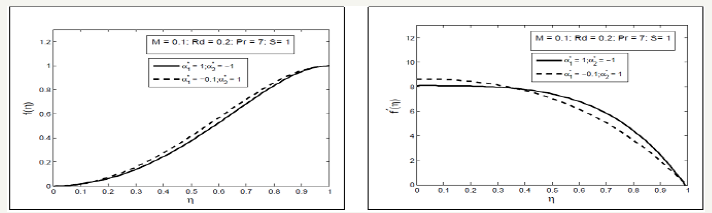Figure 14:Comparison of second grade and second order fluid for the temperature and concentration profile.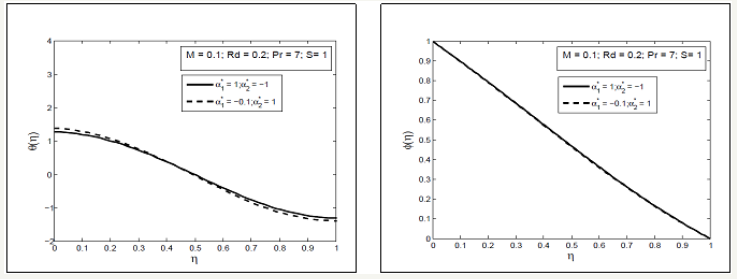#### Conclusion

In this paper we have presented a numerical approach to study heat source and chemical reaction of radiative unsteady MHD squeezed flow of second order fluid under the effect of thermal boundary conditions. The axisymmetric squeezed flow is induced by time dependent motion of parallel plates. Radiative squeezed flow of Newtonian and non-Newtonian fluids with heat source and chemical reaction under the convective boundary condition is a current topic of interest. Different studies exist in literature in which the squeezed flow in different geometries discussed [19,21,24,25].

In the present study we have concluded the following observations

a. Effect of squeezing parameter S on axial velocity f (η ) is opposite to that of second order fluid parameters and Hartmann number M .

b. Large values of squeezing parameter S second order fluid parameters and magnetic parameter M on radial velocity f ′(η ) shows the dual behavior.

c. Temperature distribution shows the mixed behavior for larger heat source Q , squeezed number S , Prandtl number Pr and thermal slip parameter

d. Mass concentration increases by increasing squeezing parameter S and chemical reaction parameters

e. Skin friction coefficient decreases for higher values of magnetic parameter M whereas increases for large values of squeezing parameter S

f. Nusselt number ( Nu ) enhances for large values of heat source parameter Q and Prandtl number Pr but decreases for large values of thermal slip parameter γ .

g. Sherwood number ( Sh ) accelerate for large values of Schmidt number Sc and decelerate for the large values of chemical reaction parameter R1

h. Pressure on the upper plate increases in negative direction with the increase in the fluid parameter and squeezing parameter S .

i. Axial velocity is greater for the second order fluid as compared with the second grade fluid while radial velocity for the second order fluid is greater near the lower plate but smaller near the upper plate as compared with the second grade fluid.

j. Temperature profile near the lower plate is greater in second order fluid as compared with the second grade fluid while reverse near the upper plate.

k. Concentration profile is same for both second grade and second order fluid.

© 2018 Mann AB . This is an open access article distributed under the terms of the Creative Commons Attribution License , which permits unrestricted use, distribution, and build upon your work non-commercially.

We at Crimson Publishing are a group of people with a combined passion for science and research, who wants to bring to the world a unified platform where all scientific know-how is available read more...